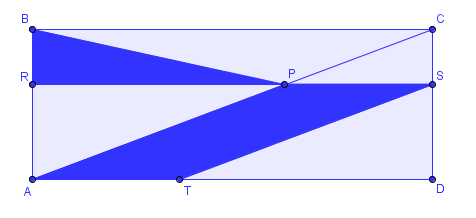# A New Flag?

Geometry Level 2

$ABCD$ is a rectangle and $P$ is any point on $AC$ (except $A$ and $C$). Through $P$ is plotted a line parallel to $BC$ which cuts $AB$ and $DC$ at $R$ and $S$, respectively. Also, through $S$ is plotted a line parallel to $AC$ which cuts $AD$ at $T$.

Find $\dfrac{\text{Area }TSPA}{\text{Area } PRB}$.×

Problem Loading...

Note Loading...

Set Loading...